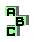CIRCUMCIRCLE    (11/13)

 r1631 altitude feet, Gob point, tangent circles r1657 altitudes, De Longchamps point r1658 altitude feet, centroid r1659 altitudes, circumcenter, Euler line r1660 tripole, symmedian point, inconic, perspector r1676 anticevian triangle, Stammler hyperbola, inverse points r1677 ninecircle, isogonal points, inverse points r1678 cevian triangle, orthologic triangles, inverse points r1685 circumconic, similar triangles r1686 centroid, similar triangles r1688 circumconic, point X(115), De Longchamps point, Tarry point, inverse points r1691 point X(100), point X(190), Steiner circumellipse r1717 circumcenter r1720 isogonal points, perspective r1732 excircles, orthic triangle, Clawson point, homothetic triangles r1734 excircles, perspective, medial triangle, Spieker point r1735 excircles, perspective, Feuerbach point r1741 altitudes, De Longchamps point r1742 altitudes, Euler line, circumcenter r1769 circumcevian triangle, tripole r1770 circumconic, perspector, circumcevian triangle, tripole r1777 Euler line r1784 circumcenter, altitudes, ninecircle r1785 orthocenter, altitudes, isogonal points, points X(1113)-X(1114) r1786 orthocenter, circumcevian triangle, medial triangle1 2 3 4 5 6 7 8 9 10 11 12 13